# NCERT Solutions for Chapter 3 Playing with Numbers Class 6 Maths### Playing with Numbers Questions and Answers

 Chapter Name Playing with Numbers NCERT Solutions Class CBSE Class 6 Textbook Name Maths Related Readings NCERT Solutions for Class 6NCERT Solutions for Class 6 Maths Summary of Playing with Numbers

Exercise 3.1

Question 1: Write all the factors of the following numbers:

(a) 24

(b) 15

(c) 21

(d) 27

(e) 12

(f) 20

(g) 18

(h) 23

(i) 36

Solution

(a) 24 = 1 × 24 = 2 × 12 = 3 × 8 = 4 × 6 = 6 × 4

∴ Factors of 24 = 1, 2, 3, 4, 6, 12, 24

(b) 15 = 1 × 15 = 3 × 5 = 5 × 3

∴ Factors of 15 = 1, 3, 5, 15

(c) 21 = 1 × 21 = 3 × 7 = 7 × 3

∴ Factors of 21 = 1, 3, 7, 21

(d) 27 = 1 × 27 = 3 × 9 = 9 × 3

∴ Factors of 27 = 1, 3, 9, 27

(e) 12 = 1 × 12 = 2 × 6 = 3 × 4 = 4 × 3

∴ Factors of 12 = 1, 2, 3, 4, 6, 12

(f) 20 = 1 × 20 = 2 × 10 = 4 × 5 = 5 × 4

∴ Factors of 20 = 1, 2, 4, 5, 10, 20

(g) 18 = 1 × 18 = 2 × 9 = 3 × 6

∴ Factors of 18 = 1, 2, 3, 6, 9, 18

(h) 23 = 1 × 23

∴ Factors of 23 = 1, 23

(i) 36 = 1 × 36 = 2 × 18 = 3 × 12 = 4 × 9 = 6 × 6

∴ Factors of 36 = 1, 2, 3, 4, 6, 9, 12, 18, 36

Question 2: Write first five multiples of:

(a) 5

(b) 8

(c) 9

Solution

(a) 5 × 1 = 5, 5 × 2 = 10, 5 × 3 = 15, 5 × 4 = 20, 5 × 5 = 25

∴ First five multiples of 5 are 5, 10, 15, 20, 25.

(b) 8 × 1 = 8, 8 × 2 = 16, 8 × 3 = 24, 8 × 4 = 32, 8 × 5 = 40

∴ First five multiples of 8 are 8, 16, 24, 32, 40.

(c) 9 × 1 = 9, 9 × 2 = 18, 9 × 3 = ,27, 9 × 4 = 36, 9 × 5 = 45

∴ First five multiples of 9 are 9, 18, 27, 36, 45

Question 3: Match the items in column 1 with the items in column 2:

 Column I Column II (i)   35 (a)  Multiple of 8 (ii)  15 (b)  Multiple of 7 (iii) 16 (c)  Multiple of 70 (iv) 20 (d)  Factor of 30 (v)  25 (e)  Factor of 50 (f)  Factor of 20

Solution

 Column I Column II (i)   35 (b)  Multiple of 7 (ii)  15 (d)  Factor of 30 (iii) 16 (a)  Multiple of 8 (iv) 20 (f)  Factor of 20 (v)  25 (e)  Factor of 50

Question 4: Find all the multiples of 9 up to 100.

Solution

Multiples of 9 up to 100 are: 9, 18, 27, 36, 45, 54, 63, 72, 81, 90, 99

Exercise 3.2

Question 1: What is the sum of any two:

(a) Odd numbers.

(b) Even numbers.

Solution

(a) The sum of any two odd numbers is an even number.

Example: 1 + 3 = 4, 3 + 5 = 8

(b) The sum of any two even numbers is also an even number.

Example: 2 + 4 = 6, 6 + 8 = 14

Question 2: State whether the following statements are true or false:

(a) The sum of three odd numbers is even.

(b) The sum of two odd numbers and one even number is even.

(c) The product of three odd numbers is odd.

(d)If an even number is divided by 2, the quotient is always odd.

(e) All prime numbers are odd.

(f) Prime numbers do not have any factors.

(g) Sum of two prime numbers is always even.

(h) 2 is the only even prime number.

(i) All even numbers are composite numbers.

(j) The product of two even numbers is always even.

Solution

(a) False

(b) True

(c) True

(d) False

(e) False

(f) False

(g) False

(h) True

(i) False

(j) True

Question 3: The numbers 13 and 31 are prime numbers. Both these numbers have same digits 1 and 3. Find such pairs of prime numbers up to 100.

Solution

17 and 71;

37 and 73;

79 and 97

Question 4: Write down separately the prime and composite numbers less than 20.

Solution

Prime numbers : 2, 3, 5, 7, 11, 13, 17, 19

Composite numbers : 4, 6, 8, 9, 10, 12, 14, 15, 16, 18

Question 5: What is the greatest prime number between 1 and 10?

Solution

The greatest prime number between 1 and 10 is ‘7’.

Question 6: Express the following as the sum of two odd numbers:

(a) 44

(b) 36

(c) 24

(d) 18

Solution

(a) 3 + 41 = 44

(b) 5 + 31 = 36

(c) 7 + 17 = 24

(d) 7 + 11 = 18

Question 7: Give three pairs of prime numbers whose difference is 2.

[Remark: Two prime numbers whose difference is 2 are called twin primes.]

Solution

3 and 5;

5 and 7;

11 and 13

Question 8: Which of the following numbers are prime:

(a) 23

(b) 51

(c) 37

(d) 26

Solution

(a) 23 and (c) 37 are prime numbers.

Question 9: Write seven consecutive composite numbers less than 100 so that there is no prime number between them.

Solution

Seven consecutive composite numbers:

90, 91, 92, 93, 94, 95, 96

Question 10: Express each of the following numbers as the sum of three odd primes:

(a) 21

(b) 31

(c) 53

(d) 61

Solution

(a) 21 = 3 + 7 + 11

(b) 31 = 3 + 11 + 17

(c) 53 = 13 + 17 + 23

(d) 61 = 19 + 29 + 13

Question 11: Write five pairs of prime numbers less than 20 whose sum is divisible by 5.

[Hint: 3 + 7 = 10]

Solution

2 + 3 = 5;

7 + 13 = 20;

3 + 17 = 20;

2 + 13 = 15;

5 + 5 = 10

Question 12: Fill in the blanks:

(a) A number which has only two factors is called a ________.

(b) A number which has more than two factors is called a _________.

(c) 1 neither ________ nor ________.

(d) The smallest prime number is _________.

(e) The smallest composite number is _________.

(f) The smallest even number is __________.

Solution

(a) Prime number

(b) Composite number

(c) Prime number and composite number

(d) 2

(e) 4

(f) 2

Exercise 3.3

Question 1: Using divisibility test, determine which of the following numbers are divisible by 2; by 3; by 4; by 5; by 6; by 8; by 9; by 10; by 11. (Say yes or no)

 Number Divisible by 2 3 4 5 6 8 9 10 11 128 990 1586 275 6686 639210 429714 2856 3060 406839

Solution

 Number Divisible by 2 3 4 5 6 8 9 10 11 128 990 1586 275 6686 639210 429714 2856 3060 406839 Yes Yes Yes No Yes Yes Yes Yes Yes No No Yes No No No Yes Yes Yes Yes Yes Yes No No No No No No Yes Yes No No Yes No Yes No Yes No No Yes No No Yes No No No Yes Yes Yes Yes No Yes No No No No No No Yes No No No Yes No No No No Yes No Yes No No Yes No No No Yes No No Yes No No Yes No Yes No Yes No No No No

Question 2: Using divisibility test, determine which of the following numbers are divisible by 4; by 8:

(a) 572

(b) 726352

(c) 5500

(d) 6000

(e) 12159

(f) 14560

(g) 21084

(h) 31795072

(i) 1700

(j) 2150

Solution

(a) 572 →Divisible by 4 as its last two digits are divisible by 4.

→Not divisible by 8 as its last three digits are not divisible by 8.

(b) 726352 →Divisible by 4 as its last two digits are divisible by 4.

→Divisible by 8 as its last three digits are divisible by 8.

(c) 5500 →Divisible by 4 as its last two digits are divisible by 4.

→Not divisible by 8 as its last three digits are not divisible by 8.

(d) 6000 →Divisible by 4 as its last two digits are 0.

→Divisible by 8 as its last three digits are 0.

(e) 12159 →Not divisible by 4 and 8 as it is an odd number.

(f) 14560 →Divisible by 4 as its last two digits are divisible by 4.

→Divisible by 8 as its last three digits are divisible by 8.

(g) 21084 →Divisible by 4 as its last two digits are divisible by 4.

→Not divisible by 8 as its last three digits are not divisible by 8.

(h) 31795072 →Divisible by 4 as its last two digits are divisible by 4.

→Divisible by 8 as its last three digits are divisible by 8.

(i) 1700 →Divisible by 4 as its last two digits are 0.

→Not divisible by 8 as its last three digits are not divisible by 8.

(j) 5500 →Not divisible by 4 as its last two digits are not divisible by 4.

→Not divisible by 8 as its last three digits are not divisible by 8.

Question 3: Using divisibility test, determine which of the following numbers are divisible by 6:

(a) 297144

(b) 1258

(c) 4335

(d) 61233

(e) 901352

(f) 438750

(g) 1790184

(h) 12583

(i) 639210

(j) 17852

Solution

(a) 297144 →Divisible by 2 as its units place is an even number.

→Divisible by 3 as sum of its digits (= 27) is divisible by 3.

Since the number is divisible by both 2 and 3, therefore, it is also divisible by 6.

(b) 1258 →Divisible by 2 as its units place is an even number.

→Not divisible by 3 as sum of its digits (= 16) is not divisible by 3.

Since the number is not divisible by both 2 and 3, therefore, it is not divisible by 6.

(c) 4335 →Not divisible by 2 as its units place is not an even number.

→Divisible by 3 as sum of its digits (= 15) is divisible by 3.

Since the number is not divisible by both 2 and 3, therefore, it is not divisible by 6.

(d) 61233 →Not divisible by 2 as its units place is not an even number.

→Divisible by 3 as sum of its digits (= 15) is divisible by 3.

Since the number is not divisible by both 2 and 3, therefore, it is not divisible by 6.

(e) 901352 →Divisible by 2 as its units place is an even number.

→Not divisible by 3 as sum of its digits (= 20) is not divisible by 3.

Since the number is not divisible by both 2 and 3, therefore, it is not divisible by 6.

(f) 438750 →Divisible by 2 as its units place is an even number.

→Divisible by 3 as sum of its digits (= 27) is not divisible by 3.

Since the number is divisible by both 2 and 3, therefore, it is divisible by 6.

(g) 1790184 →Divisible by 2 as its units place is an even number.

→Divisible by 3 as sum of its digits (= 30) is not divisible by 3.

Since the number is divisible by both 2 and 3, therefore, it is divisible by 6.

(h) 12583 →Not divisible by 2 as its units place is not an even number.

→Not divisible by 3 as sum of its digits (= 19) is not divisible by 3.

Since the number is not divisible by both 2 and 3, therefore, it is not divisible by 6.

(i) 639210 →Divisible by 2 as its units place is an even number.

→Divisible by 3 as sum of its digits (= 21) is not divisible by 3.

Since the number is divisible by both 2 and 3, therefore, it is divisible by 6.

(j) 17852 →Divisible by 2 as its units place is an even number.

→Not divisible by 3 as sum of its digits (= 23) is not divisible by 3.

Since the number is not divisible by both 2 and 3, therefore, it is not divisible by 6.

Question 4: Using divisibility test, determine which of the following numbers are divisible by 11:

(a) 5445

(b) 10824

(c) 7138965

(d) 70169308

(e) 10000001

(f) 901153

Solution

(a) 5445 →Sum of the digits at odd places = 4 + 5 = 9

→Sum of the digits at even places = 4 + 5 = 9

→Difference of both sums = 9 – 9 = 0

Since the difference is 0, therefore, the number is divisible by 11.

(b) 10824 →Sum of the digits at odd places = 4 + 8 +1 = 13

→Sum of the digits at even places = 2 + 0 = 2

→Difference of both sums = 13 – 2 = 11

Since the difference is 11, therefore, the number is divisible by 11.

(c) 7138965 →Sum of the digits at odd places = 5 + 9 + 3 + 7 = 24

→Sum of the digits at even places = 6 + 8 + 1 = 15

→Difference of both sums = 24 – 15 = 9

Since the difference is neither 0 nor 11, therefore, the number is not divisible by 11.

(d) 70169308 →Sum of the digits at odd places = 8 + 3 + 6 + 0 = 17

→Sum of the digits at even places = 0 + 9 + 1 + 7 = 17

→Difference of both sums = 17 – 17 = 0

Since the difference is 0, therefore, the number is divisible by 11.

(e) 10000001 →Sum of the digits at odd places = 1 + 0 + 0 + 0 = 1

→Sum of the digits at even places = 0 + 0 + 0 + 1 = 1

→Difference of both sums = 1 – 1 = 0

Since the difference is 0, therefore, the number is divisible by 11.

(f) 901153 →Sum of the digits at odd places = 3 + 1 + 0 = 4

→Sum of the digits at even places = 5 + 1 + 9 = 15

→Difference of both sums = 15 – 4 = 11

Since the difference is 11, therefore, the number is divisible by 11.

Question 5: Write the smallest digit and the largest digit in the blanks space of each of the following numbers so that the number formed is divisibly by 3:

(a) __ 6724

(b) 4765 __ 2

Solution

(a) We know that a number is divisible by 3 if the sum of all digits is divisible by 3.

Therefore, Smallest digit : 2 →26724 = 2 + 6 + 7 + 2 + 4 = 21

Largest digit : 8 →86724 = 8 + 6 + 7 + 2 + 4 = 27

(b) We know that a number is divisible by 3 if the sum of all digits is divisible by 3.

Therefore, Smallest digit : 0 →476502 = 4 + 7 + 6 + 5 + 0 + 2 = 24

Largest digit : 9 →476592 = 4 + 7 + 6 + 5 + 0 + 2 = 33

Question 6: Write a digit in the blanks space of each of the following numbers so that the number formed is divisible by 11:

(a) 92 __ 389

(b) 8 __ 9484

Solution

(a) We know that a number is divisible by 11 if the difference of the sum of the digits at odd places and that of even places should be either 0 or 11.

Therefore, 928389 →Odd places = 9 + 8 + 8 = 25

Even places = 2 + 3 + 9 = 14

Difference = 25 – 14 = 11

(b) We know that a number is divisible by 11 if the difference of the sum of the digits at odd places and that of even places should be either 0 or 11.

Therefore, 869484 →Odd places = 8 + 9 + 8 = 25

Even places = 6 + 4 + 4 = 14

Difference = 25 – 14 = 11

Exercise 3.4

Question 1: Find the common factors of:

(a) 20 and 28

(b) 15 and 25

(c) 35 and 50

(d) 56 and 120

Solution

(a) Factors of 20 = 1, 2, 4, 5, 10, 20

Factors of 28 = 1, 2, 4, 7, 14, 28

Common factors = 1, 2, 4

(b) Factors of 15 = 1, 3, 5, 15

Factors of 25 = 1, 5, 25

Common factors = 1, 5

(c) Factors of 35 = 1, 5, 7, 35

Factors of 50 = 1, 2, 5, 10, 25, 50

Common factors = 1, 5

(d) Factors of 56 = 1, 2, 4, 7, 8, 14, 28, 56

Factors of 120 = 1, 2, 3, 4, 5, 6, 8, 10, 12, 15, 20, 24, 30, 60, 120

Common factors = 1, 2, 4, 8

Question 2: Find the common factors of:

(a) 4, 8 and 12

(b) 5, 15 and 25

Solution

(a) Factors of 4 = 1, 2, 4

Factors of 8 = 1, 2, 4, 8

Factors of 12 = 1, 2, 3, 4, 6, 12

Common factors of 4, 8 and 12 = 1, 2, 4

(b) Factors of 5 = 1, 5

Factors of 15 = 1, 3, 5, 15

Factors of 25 = 1, 5, 25

Common factors of 5, 15 and 25 = 1, 5

Question 3: Find the first three common multiples of:

(a) 6 and 8

(b) 12 and 18

Solution

(a) Multiple of 6 = 6, 12, 18, 24, 30, 36, 42, 28, 54, 60, 72, ...............

Multiple of 8 = 8, 16, 24, 32, 40, 48, 56, 64, 72, ..............

Common multiples of 6 and 8 = 24, 48, 72

(b) Multiple of 12 = 12, 24, 36, 48, 60, 72, 84, 96, 108, 120, ...............

Multiple of 18 = 18, 36, 54, 72, 90, 108, ................

Common multiples of 12 and 18 = 36, 72, 108

Question 4: Write all the numbers less than 100 which are common multiples of 3 and 4.

Solution

Multiple of 3 = 3, 6, 9, 12, 15, 18, 21, 24, 27, 30, 33, 36, 39, 42, 45, 48, 51, 54, 57, 60, 63, 66, 69, 72, 75, 78, 81, 84, 87, 90, 93, 96, 99

Multiple of 4 = 4, 8, 12, 16, 20, 24, 28, 32, 36, 40, 44, 48, 52, 56, 60, 64, 68, 72, 76, 80, 84, 88, 92, 96, 100

Common multiples of 3 and 4 less than 100 = 12, 24, 36, 48, 60, 72, 84, 96

Question 5: Which of the following numbers are co-prime:

(a) 18 and 35

(b) 15 and 37

(c) 30 and 415

(d) 17 and 68

(e) 216 and 215

(f) 81 and 16

Solution

(a) Factors of 18 = 1, 2, 3, 6, 9, 18

Factors of 35 = 1, 5, 7, 35

Common factor = 1

Since, both have only one common factor, i.e., 1, therefore, they are co-prime numbers.

(b) Factors of 15 = 1, 3, 5, 15

Factors of 37 = 1, 37

Common factor = 1

Since, both have only one common factor, i.e., 1, therefore, they are co-prime numbers.

(c) Factors of 30 = 1, 2, 3, 5, 6, 15, 30

Factors of 415 = 1, 5, 83, 415

Common factor = 1, 5

Since, both have more than one common factor, therefore, they are not co-prime numbers.

(d) Factors of 17 = 1, 17

Factors of 68 = 1, 2, 4, 17, 34, 86

Common factor = 1, 17

Since, both have more than one common factor, therefore, they are not co-prime numbers.

(e) Factors of 216 = 1, 2, 3, 4, 6, 8, 36, 72, 108, 216

Factors of 215 = 1, 5, 43, 215

Common factor = 1

Since, both have only one common factor, i.e., 1, therefore, they are co-prime numbers.

(f) Factors of 81 = 1, 3, 9, 27, 81

Factors of 16 = 1, 2, 4, 8, 16

Common factor = 1

Since, both have only one common factor, i.e., 1, therefore, they are co-prime numbers.

Question 6: A number is divisible by both 5 and 12. By which other number will that number be always divisible?

Solution

× 12 = 60. The number must be divisible by 60.

Question 7: A number is divisible by 12. By what other numbers will that number be divisible?

Solution

Factors of 12 are 1, 2, 3, 4, 6 and 12.

Therefore, the number also be divisible by 1, 2, 3 4 and 6.

Exercise 3.5

Question 1: Which of the following statements are true:

(a) If a number is divisible by 3, it must be divisible by 9.

(b)If a number is divisible by 9, it must be divisible by 3.

(c) If a number is divisible by 18, it must be divisible by both 3 and 6.

(d)If a number is divisible by 9 and 10 both, then it must be divisible by 90.

(e) If two numbers are co-primes, at least one of them must be prime.

(f) All numbers which are divisible by 4 must also by divisible by 8.

(g) All numbers which are divisible by 8 must also by divisible by 4.

(h)If a number is exactly divides two numbers separately, it must exactly divide their sum.

(i) If a number is exactly divides the sum of two numbers, it must exactly divide the two numbers separately.

Solution

Statements (b), (c), (d), (g) and (h) are true.

Question 2: Here are two different factor trees for 60. Write the missing numbers.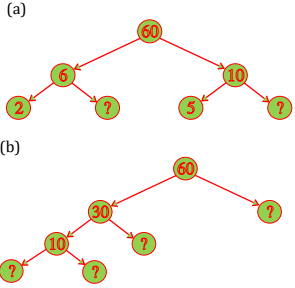Solution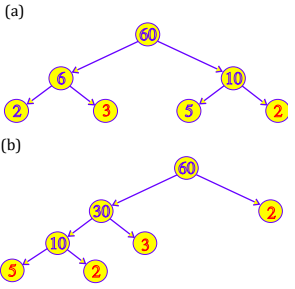Question 3: Which factors are not included in the prime factorization of a composite number?

Solution

1 is the factor which is not included in the prime factorization of a composite number.

Question 4: Write the greatest 4-digit number and express it in terms of its prime factors.

Solution

The greatest 4-digit number = 9999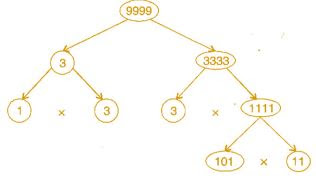The prime factors of 9999 are 3 × 3 × 11 × 101

Question 5: Write the smallest 5-digit number and express it in terms of its prime factors.

Solution

The smallest five digit number is 10000.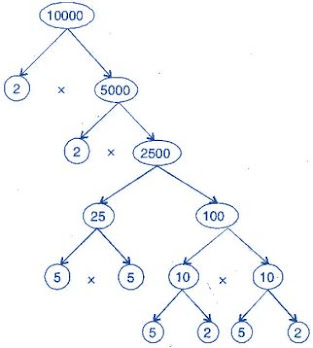The prime factors of 10000 are 2 × 2 × 2 × 2 × 5 × 5 × 5 × 5.

Question 6: Find all the prime factors of 1729 and arrange them in ascending order. Now state the relation, if any, between, two consecutive prime numbers.

Solution

Prime factors of 1729 are 7 × 13 × 19.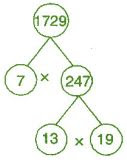The difference of two consecutive prime factors is 6.

Question 7: The product of three consecutive numbers is always divisible by 6. Verify this statement with the help of some examples.

Solution

Among the three consecutive numbers, there must be one even number and one multiple of 3. Thus, the product must be multiple of 6.

Example: (i) 2 × 3 × 4 = 24

(ii) 4 × 5 × 6 = 120

Question 8: The sum of two consecutive odd numbers is always divisible by 4. Verify this statement with the help of some examples.

Solution

3 + 5 = 8 and 8 is divisible by 4.

5 + 7 = 12 and 12 is divisible by 4.

7 + 9 = 16 and 16 is divisible by 4.

9 + 11 = 20 and 20 is divisible by 4.

Question 9: In which of the following expressions, prime factorization has been done:

(a) 24 = 2 × 3 × 4

(b) 56 = 7 × 2 × 2 × 2

(c) 70 = 2 × 5 × 7

(d) 54 = 2 × 3 × 9

Solution

In expressions (b) and (c), prime factorization has been done.

Question 10: Determine if 25110 is divisible by 45.

[Hint: 5 and 9 are co-prime numbers. Test the divisibility of the number by 5 and 9.]

Solution

The prime factorization of 45 = 5 × 9

25110 is divisible by 5 as ‘0’ is at its unit place.

25110 is divisible by 9 as sum of digits is divisible by 9.

Therefore, the number must be divisible by 5 × 9 = 45

Question 11: 18 is divisible by both 2 and 3. It is also divisible by 2 × 3 = 6. Similarly, a number is divisible by 4 and 6. Can we say that the number must be divisible by 4 × 6 = 24? If not, give an example to justify your answer.

Solution

No. Number 12 is divisible by both 6 and 4 but 12 is not divisible by 24.

Question 12: I am the smallest number, having four different prime factors. Can you find me?

Solution

The smallest four prime numbers are 2, 3, 5 and 7.

Hence, the required number is 2 × 3 × 5 × 7 = 210

Exercise 3.6

Question 1: Find the H.C.F. of the following numbers:

(a) 18, 48

(b) 30, 42

(c) 18, 60

(d) 27, 63

(e) 36, 84

(f) 34, 102

(g) 70, 105, 175

(h) 91, 112, 49

(i) 18, 54, 81

(j) 12, 45, 75

Solution

(a) Factors of 18 = 2 × 3 × 3

Factors of 48 = 2 × 2 × 2 × 2 × 3

H.C.F. (18, 48) = 2 × 3 = 6

(b) Factors of 30 = 2 × 3 × 5

Factors of 42 = 2 × 3 × 7

H.C.F. (30, 42) = 2 × 3 = 6

(c) Factors of 18 = 2 × 3 × 3

Factors of 60 = 2 × 2 × 3 × 5

H.C.F. (18, 60) = 2 × 3 = 6

(d) Factors of 27 = 3 × 3 × 3

Factors of 63 = 3 × 3 × 7

H.C.F. (27, 63) = 3 × 3 = 9

(e) Factors of 36 = 2 × 2 × 3 × 3

Factors of 84 = 2 × 2 × 3 × 7

H.C.F. (36, 84) = 2 × 2 × 3 = 12

(f) Factors of 34 = 2 × 17

Factors of 102 = 2 × 3 × 17

H.C.F. (34, 102) = 2 × 17 = 34

(g) Factors of 70 = 2 × 5 × 7

Factors of 105 = 3 × 5 × 7

Factors of 175 = 5 × 5 × 7

H.C.F. = 5 × 7 = 35

(h) Factors of 91 = 7 × 13

Factors of 112 = 2 × 2 × 2 × 2 × 7

Factors of 49 = 7 × 7

H.C.F. = 1 × 7 = 7

(i) Factors of 18 = 2 × 3 × 3

Factors of 54 = 2 × 3 × 3 × 3

Factors of 81 = 3 × 3 × 3 × 3

H.C.F. = 3 × 3 = 9

(j) Factors of 12 = 2 × 2 × 3

Factors of 45 = 3 × 3 × 5

Factors of 75 = 3 × 5 × 5

H.C.F. = 1 × 3 = 3

Question 2: What is the H.C.F. of two consecutive:

(a) numbers?

(b) even numbers?

(c) odd numbers?

Solution

(a) H.C.F. of two consecutive numbers be 1.

(b) H.C.F. of two consecutive even numbers be 2.

(c) H.C.F. of two consecutive odd numbers be 1.

Question 3: H.C.F. of co-prime numbers 4 and 15 was found as follows by factorization:

4 = 2 × 2 and 15 = 3 × 5 since there is no common prime factor, so H.C.F. of  4 and 15 is 0. Is the answer correct? If not, what is the correct H.C.F.?

Solution

No. The correct H.C.F. is 1.

Exercise 3.7

Question 1: Renu purchases two bags of fertilizer of weights 75 kg and 69 kg. Find the maximum value of weight which can measure the weight of the fertilizer exact number of times.

Solution

For finding maximum weight, we have to find H.C.F. of 75 and 69.

Factors of 75 = 3 × 5 × 5

Factors of 69 = 3 × 69

H.C.F. = 3

Therefore the required weight is 3 kg.

Question 2: Three boys step off together from the same spot. Their steps measure 63 cm, 70 cm and 77 cm respectively. What is the maximum distance each should cover so that all can cover the distance in complete steps?

Solution

For finding minimum distance, we have to find L.C.M of 63, 70 and 77.

 7 63,70,77 9 9,10,11 10 1,10,11 11 1,1,11 1,1,1

L.C.M. of 63, 70 and 77 = 7 × 9 × 10 × 11 = 6930 cm.

Therefore, the minimum distance is 6930 cm.

Question 3: The length, breadth and height of a room are 825 cm, 675 cm and 450 cm respectively.

Find the longest tape which can measure the three dimensions of the room exactly.

Solution

The measurement of longest tape = H.C.F. of 825 cm, 675 cm and 450 cm.

Factors of 825 = 3 × 5 × 5 × 11

Factors of 675 = 3 × 5 × 5 × 3 × 3

Factors of 450 = 2 × 3 × 3 × 5 × 5

H.C.F. = 3 × 5 × 5 = 75 cm

Therefore, the longest tape is 75 cm.

Question 4: Determine the smallest 3-digit number which is exactly divisible by 6, 8 and 12.

Solution

 2 6, 8, 12 2 3, 4, 6 2 3, 2, 3 3 3, 1, 3 1, 1, 1

L.C.M. of 6, 8 and 12 = 2 × 2 × 2 × 3 = 24

The smallest 3-digit number = 100

To find the number, we have to divide 100 by 24

100 = 24 × 4 + 4

Therefore, the required number = 100 + (24 – 4) = 120.

Question 5: Determine the largest 3-digit number which is exactly divisible by 8, 10 and 12.

Solution

 2 8, 10, 12 2 4, 5, 6 2 2, 5, 3 3 1, 5, 3 5 1, 5, 1 1, 1, 1

L.C.M. of 8, 10, 12 = 2 × 2 × 2 × 3 × 5 = 120

The largest three digit number = 999

Now,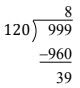Therefore, the required number = 999 – 39 = 960

Question 6: The traffic lights at three different road crossings change after every 48 seconds, 72 seconds and 108 seconds respectively. If they change simultaneously at 7 a.m. at what time will they change simultaneously again?

Solution

 2 48, 72, 108 2 24, 36, 54 2 12, 18,  27 2 6, 9, 27 3 3, 9, 27 3 1, 3, 9 3 1, 1, 3 1, 1, 1

L.C.M. of 48, 72, 108 = 2 × 2 × 2 × 2 × 3 × 3 × 3 = 432 sec.

After 432 seconds, the lights change simultaneously.

432 second = 7 minutes 12 seconds

Therefore the time = 7 a.m. + 7 minutes 12 seconds

= 7:07:12 a.m.

Question 7: Three tankers contain 403 litres and 465 litres of diesel respectively. Find the maximum capacity of a container that can measure the diesel of three containers exact number of times.

Solution

The maximum capacity of container = H.C.F. (403, 434, 465)

Factors of 403 = 13 × 31

Factors of 434 = 2 × 7 × 31

Factors of 465 = 3 × 5 × 31

H.C.F. = 31

Therefore, 31 litres of container is required to measure the quantity.

Question 8: Find the least number which when divided by 6, 15 and 18, leave remainder 5 in each case.

Solution

 2 6, 15, 18 3 3, 15, 9 3 1, 5, 3 5 1, 5, 1 1, 1, 1

L.C.M. of 6, 15 and 18 = 2 × 3 × 3 × 5 = 90

Therefore, the required number = 90 + 5 = 95

Question 9: Find the smallest 4-digit number which is divisible by 18, 24 and 32.

Solution

 2 18, 24, 32 2 9, 12, 16 2 9, 6, 8 2 9, 3, 4 2 9, 3, 2 3 9, 3, 1 3 3, 1, 1 1, 1, 1

L.C.M. of 18, 24 and 32 = 2 × 2 × 2 × 2 × 2 × 3 × 3 = 288

The smallest four-digit number = 1000

Now,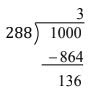Therefore, the required number is 1000 + (288 – 136) = 1152.

Question 10: Find the L.C.M. of the following numbers:

(a) 9 and 4

(b) 12 and 5

(c) 6 and 5

(d) 15 and 4

Observe a common property in the obtained L.C.Ms. Is L.C.M. the product of two numbers in each case?

Solution

(a) L.C.M. of 9 and 4

 2 9, 4 2 9, 2 3 9, 1 3 3, 1 1, 1

= 2 × 2 × 3 × 3

= 36

(b) L.C.M. of 12 and 5

 2 12, 5 2 6, 5 3 3, 5 3 1, 5 1, 1

= 2 × 2 × 3 × 5

= 60

(c) L.C.M. of 6 and 5

 2 6, 5 3 3, 5 5 1, 5 1, 1

= 2 × 3 × 5

= 30

(d) L.C.M. of 15 and 4

 2 15, 4 2 15, 2 3 15, 1 5 5, 1 1, 1

= 2 × 2 × 3 × 5

= 60

Yes, the L.C.M. is equal to the product of two numbers in each case.

And L.C.M. is also the multiple of 3.

Question 11: Find the L.C.M. of the following numbers in which one number is the factor of other:

(a) 5, 20

(b) 6, 18

(c) 12, 48

(d) 9, 45

What do you observe in the result obtained?

Solution

(a) L.C.M. of 5 and 20

 2 5, 20 2 5, 10 5 5, 5 1, 1

= 2 × 2 × 5

= 20

(b) L.C.M. of 6 and 18

 2 6, 10 3 3, 9 3 1, 3 1, 1

= 2 × 3 × 3

= 18

(c) L.C.M. of 12 and 48

 2 12, 48 2 6, 24 2 3, 12 2 3, 6 3 3, 3 1, 1

= 2 × 2 × 2 × 2 × 3

= 48

(d) L.C.M. of 9 and 45

 3 9, 45 3 3, 15 5 1, 5 1, 1

= 3 × 3 × 5

= 45

From these all cases, we can conclude that if the smallest number if the factor of largest number, then the L.C.M. of these two numbers is equal to that of larger number.